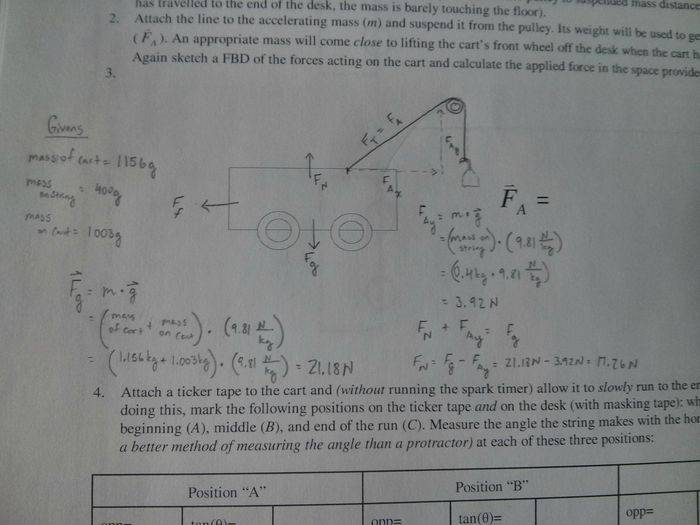# Unbalanced Force Components and Acceleration

## Homework Statement

Givens:
Mass of Cart = 1156g = 1.156kg
Mass on string = 400g = 0.4kg
Mass on Cart = 1003g = 1.003kg

The weight attached to the pulley generates the applied force.## Homework Equations

Calculate the force applied.

## The Attempt at a Solution

Look on the sheet.

Last edited:

lightgrav
Homework Helper
the Normal Force by the track is to keep the cart & load from sinking into the track.
it looks like the string is pulling somewhat upward but not entirely upward;
only the upward component will help the track not get sunk into.
Do you know that string's angle?

From a theory perspective: how much total mass is going to accelerate? (no, not 2.159kg)
which component of the string's Tension will be causing that acceleration?

Experimental perspective: did you measure its acceleration with the ticker tape?
then the horizontal component of string Force applied to (car & load) is pretty easy to deduce.

The theory, on the other hand, is a bear because the angle makes the hanger's acceleration different than the car & load acceleration.